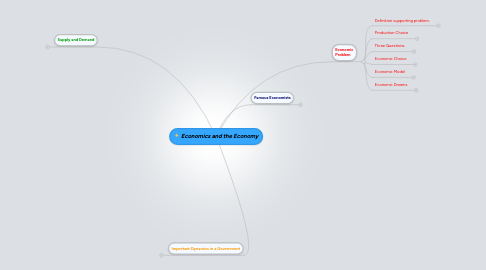# Economics and the Economy

Get Started. It's FreeEconomics and the Economy## 1. Famous Economists

1.1.1. Explained how the division of labour increases production

1.1.2. Argued that self interest is transformed by the invisible hand of competition so that it creates significant economic benefits.

1.1.3. Stressed the principle of laissez faire, which means that governments should not intervene in economic activity.

### 1.2. William Stanley Jevons

1.2.1. assumed measurable utility

1.2.2. outlined the law of diminishing marginal utility which states that a consumer’s marginal utility declines as more of a product is consumed

1.2.3. New node

## 2. Economic Problem

### 2.1. Definition supporting problem:

2.1.1. Economics is the examination of how to categorize little resources against rival ends.

2.1.1.1. Microeconomics

2.1.1.2. Macroeconomics

2.1.1.2.1. Public view of an economy

2.1.2. Humans wants are very high, but all of our economic resources cannot fulfill peoples wants. (Humans in general, want-need more than they clearly need.)

### 2.2. Production Choice

2.2.1. Possibilities Model

2.2.1.1. Propositions:

2.2.1.1.1. Economy only makes two types of products.

2.2.1.1.2. Resources and technology are stable.

2.2.1.1.3. All resources are active to their most volume.

2.2.1.2. Possibility Curve:

2.2.1.2.1. Range of possible output combinations for an economy.

2.2.1.2.2. It highlights the scarcity of resources.

2.2.1.2.3. New node

### 2.3. Three Questions:

2.3.1. What to produce?

2.3.2. How To Produce?

2.3.3. And Whom?

### 2.4. Economic Choice

2.4.1. Economists assume that economic decision- makers maximize their own utility.ew node

2.4.2. Accept economic judgement makers dismissal of their own utility.

2.4.3. Opportunity cost is defined as the utility of the best forgone alternative.

### 2.5. Economic Model

2.5.1. Shows how variables impact independent variables

2.5.2. Includes inverse and immediate associations.

2.5.3. Incorporate a variety of assumptions such as ceteris paribus

### 2.6. Economic Dreams

2.6.1. Economic efficiency

2.6.2. Price stability

2.6.3. Income equity

2.6.4. Full employment

2.6.5. Economic growth

2.6.6. Environmental sustainability

2.6.7. Achievable balance of payments

## 3. Supply and Demand

### 3.1. Demand:

3.1.1. New node

3.1.1.1. Demand is a relationship between a product’s price and quantity demanded.

3.1.2. The law of demand states that price and quantity demanded are inversely related. Market demand is the sum of quantities demanded by all consumers in a market.

3.1.3. Demand is shown using a schedule or curve.

3.1.4. Demand Determinants:

3.1.4.2. Income

3.1.4.3. Preference of the Consumer

3.1.4.4. Prices of Product (Substitute and complementary)

3.1.4.5. Expectations of the Consumers

### 3.2. Market Equilibrium

3.2.1. Shortage

3.2.1.1. there is excess demand

3.2.1.2. The price is pushed up

3.2.2. fghfg

3.2.3. Surplus

3.2.3.1. When a product is in surplus price is pushed down

3.2.3.2. there is excess supply

### 3.3. Supply

3.3.1. Supply is the relationship between a product’s price and quantity supplied

3.3.2. The law of supply states there is a direct relationship between price and quantity supplied.

3.3.3. Supply Determinants

3.3.3.1. Number of Producers

3.3.3.2. Resource Prices

3.3.3.3. State of technology

3.3.3.4. Prices of related products

3.3.3.5. Changes in nature

3.3.3.6. Producer expectations

3.3.4. Differences in quantity supplied are fit to price changes.

## 4. Important Dynamics in a Government

### 4.1. Price Controls

4.1.1. A price limitation is the most price set below the equilibrium price, this would result in a shortage.

4.1.1.1. Rent controls are a perfect example of a price limitation.

4.1.2. A price floor is a lowest price set above the equilibrium price. Which would eventually result in a surplus.

4.1.2.1. Price aids for agricultural goods are an example of a price floor

### 4.2. Price Elasticity (Demand)

4.2.1. Determinants

4.2.1.1. portion of consumer incomes (products with smaller portions more inelastic)

4.2.1.3. necessities versus luxuries (more inelastic for necessities and more elastic for luxuries)

4.2.1.4. Time (More elastic with passage of time)

4.2.2. Elastic Demand

4.2.2.1. Shows how responsive consumers are to price change

4.2.2.2. New node

4.2.2.3. Means % change in quantity demanded is more than % change in price

4.2.2.4. A price change causes total revenue to change in the opposite direction

4.2.3. Inelastic Demand

4.2.3.1. Means % change in quantity demanded is less than % change in price

4.2.3.1.1. A price change causes total revenue to change in the same direction

4.2.3.2. New node

4.2.4. Unit-elastic Demand

4.2.4.1. Means % change in quantity demanded is equal to % change in price

4.2.4.1.1. A price change does not affect total revenue

4.2.5. Perfect Elastic Demand

4.2.5.1. Constant price and a horizontal demand curve

4.2.6. Perfect Inelastic Demand

4.2.6.1. Constant price and a vertical demand curve

4.2.7. Calculating Elasticity

4.2.7.1. ed = ΔQd ÷ average Qd Δprice ÷ average price

### 4.3. Supply

4.3.1. Measures the reaction of quantity supplied to price alters.

4.3.2. Inelastic Supply

4.3.2.1. equals: percent change in quantity supplied is less than percent change in price.

4.3.3. Elastic Supply

4.3.3.1. equals: percent alteration in quantity supplied is more than percent difference in price

4.3.3.2. New node

4.3.4. Calculating Elasticity

4.3.4.1. formula: ΔQs ÷ average Qs Δprice ÷ average price

4.3.5. Perfect Elastic Supply

4.3.5.1. refers to changeless price and a horizontal supply curve

4.3.6. Perfect Inelastic Supply

4.3.6.1. : stable quantity supplied and a vertical supply curve

4.3.7. Time

4.3.7.1. Price elasticity differs over production periods

4.3.7.1.1. Supply is perfectly inelastic in immediate run

4.3.7.1.2. Supply is either elastic or inelastic in the short run

4.3.7.1.3. Supply is perfectly elastic for a constant cost industry and very elastic for an increasing cost industry in the long run